Скачать презентацию Operations Management Waiting-Line Models Module D 1

bb988938dddddb491e0a89cbb10956fd.ppt

• Количество слайдов: 57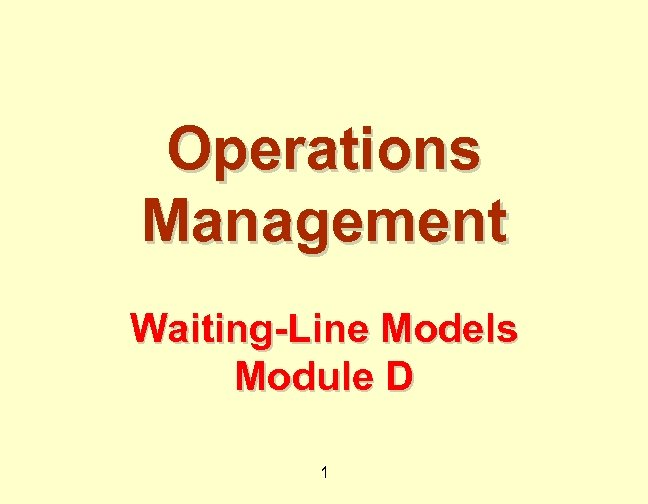Operations Management Waiting-Line Models Module D 1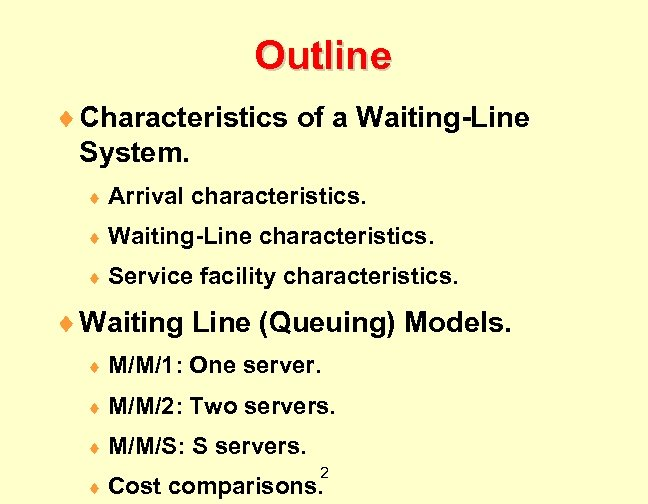Outline ¨ Characteristics of a Waiting-Line System. ¨ Arrival characteristics. ¨ Waiting-Line ¨ Service characteristics. facility characteristics. ¨ Waiting Line (Queuing) Models. ¨ M/M/1: One server. ¨ M/M/2: Two servers. ¨ M/M/S: S servers. ¨ Cost 2 comparisons.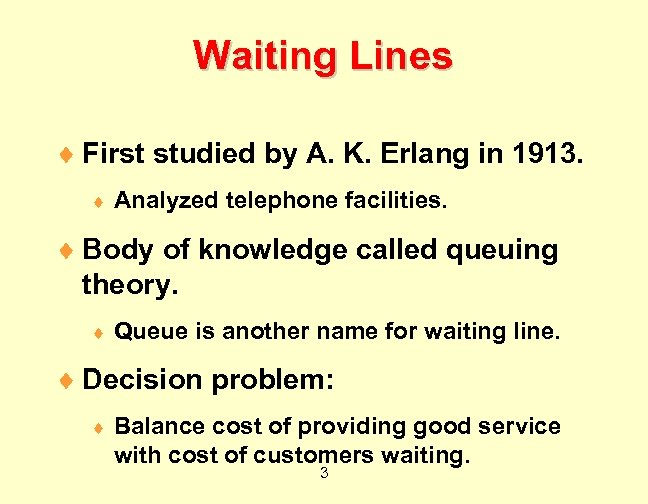Waiting Lines ¨ First studied by A. K. Erlang in 1913. ¨ Analyzed telephone facilities. ¨ Body of knowledge called queuing theory. ¨ Queue is another name for waiting line. ¨ Decision problem: ¨ Balance cost of providing good service with cost of customers waiting. 3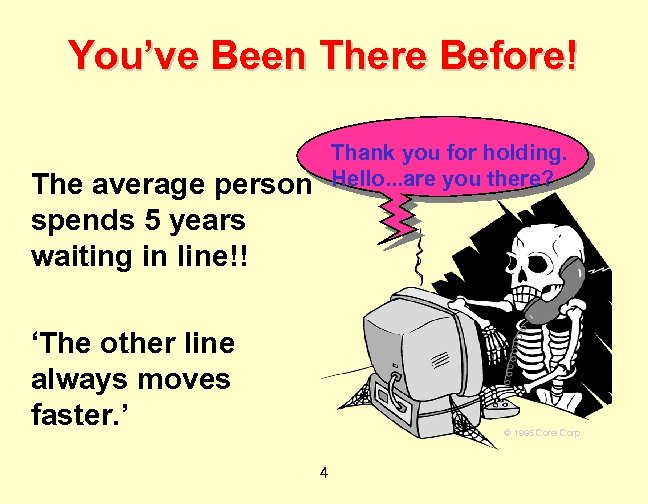You’ve Been There Before! Thank you for holding. Hello. . . are you there? The average person spends 5 years waiting in line!! ‘The other line always moves faster. ’ © 1995 Corel Corp. 4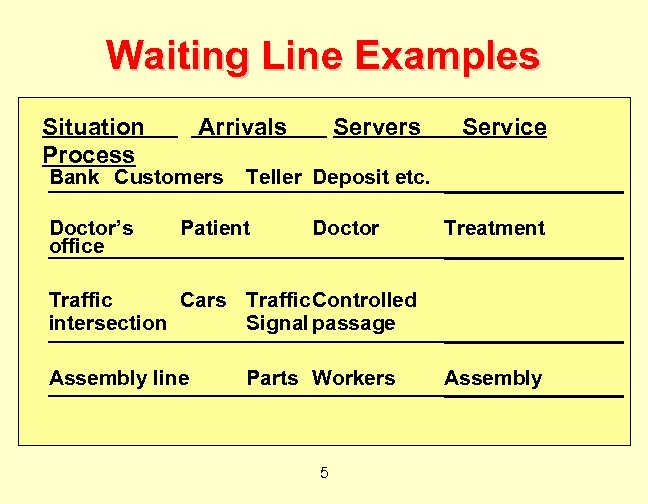Waiting Line Examples Situation Process Arrivals Bank Customers Doctor’s office Servers Service Teller Deposit etc. Patient Doctor Treatment Traffic Cars Traffic. Controlled intersection Signal passage Assembly line Parts Workers 5 Assembly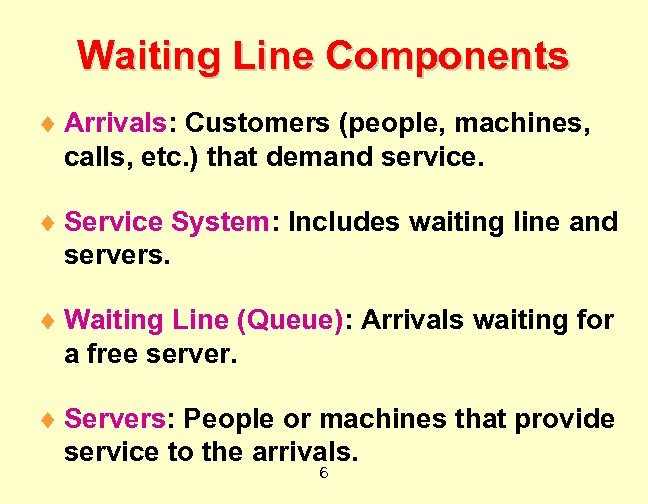Waiting Line Components ¨ Arrivals: Customers (people, machines, calls, etc. ) that demand service. ¨ Service System: Includes waiting line and servers. ¨ Waiting Line (Queue): Arrivals waiting for a free server. ¨ Servers: People or machines that provide service to the arrivals. 6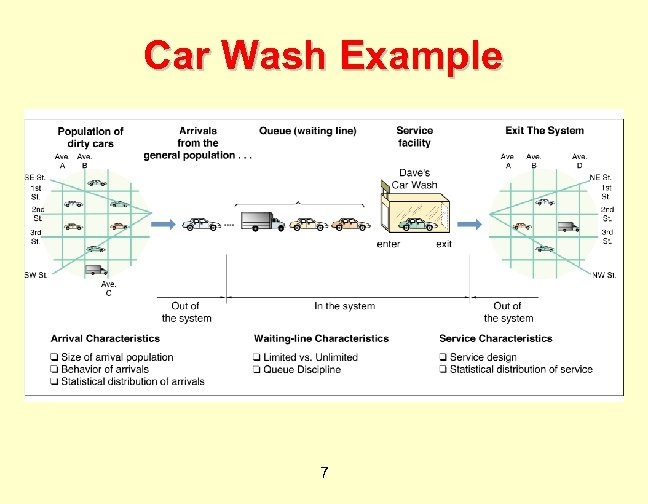Car Wash Example 7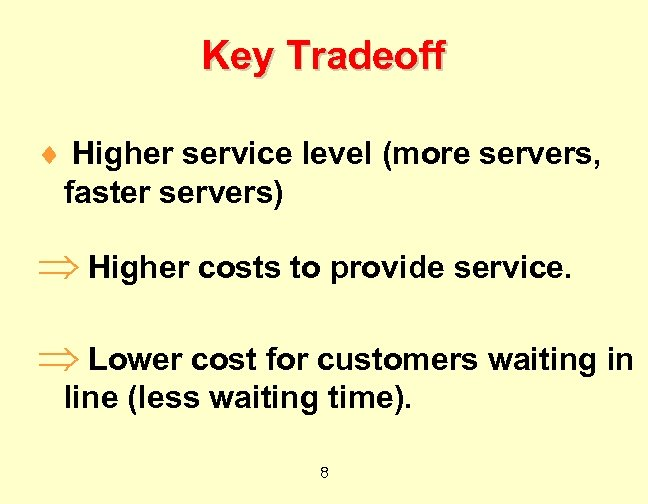Key Tradeoff ¨ Higher service level (more servers, faster servers) Þ Higher costs to provide service. Þ Lower cost for customers waiting in line (less waiting time). 8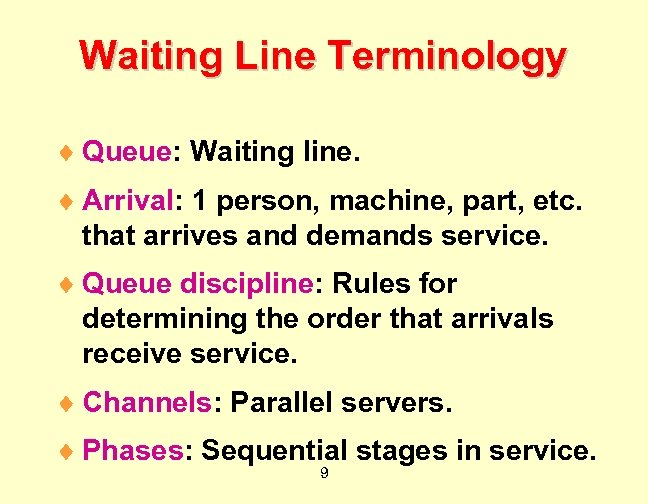Waiting Line Terminology ¨ Queue: Waiting line. ¨ Arrival: 1 person, machine, part, etc. that arrives and demands service. ¨ Queue discipline: Rules for determining the order that arrivals receive service. ¨ Channels: Parallel servers. ¨ Phases: Sequential stages in service. 9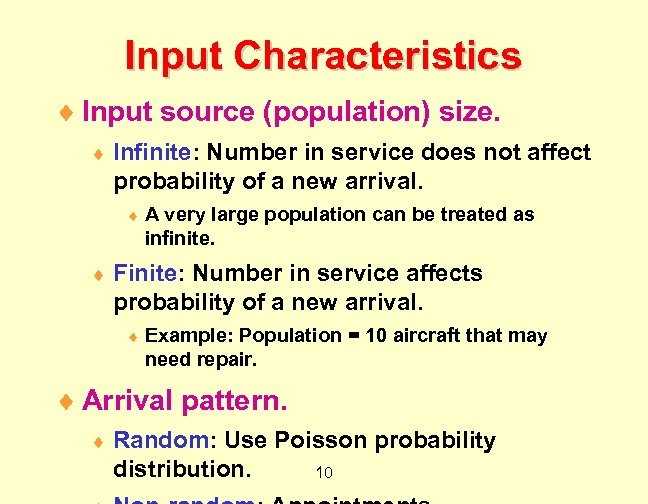Input Characteristics ¨ Input source (population) size. ¨ Infinite: Number in service does not affect probability of a new arrival. ¨ ¨ A very large population can be treated as infinite. Finite: Number in service affects probability of a new arrival. ¨ Example: Population = 10 aircraft that may need repair. ¨ Arrival pattern. ¨ Random: Use Poisson probability distribution. 10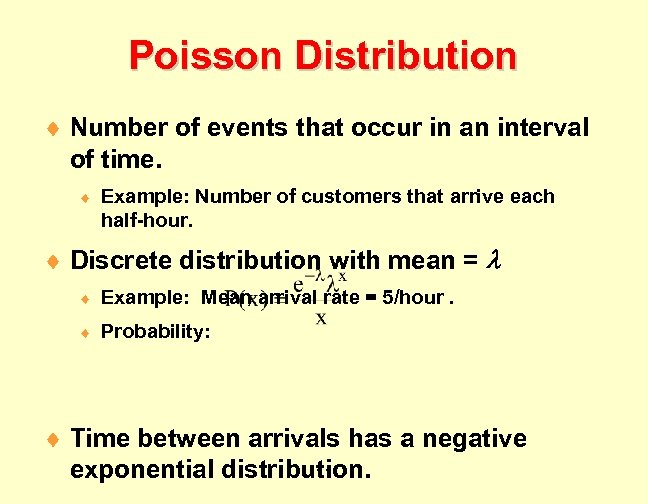Poisson Distribution ¨ Number of events that occur in an interval of time. ¨ Example: Number of customers that arrive each half-hour. ¨ Discrete distribution with mean = ¨ Example: Mean arrival rate = 5/hour. ¨ Probability: ¨ Time between arrivals has a negative 11 exponential distribution.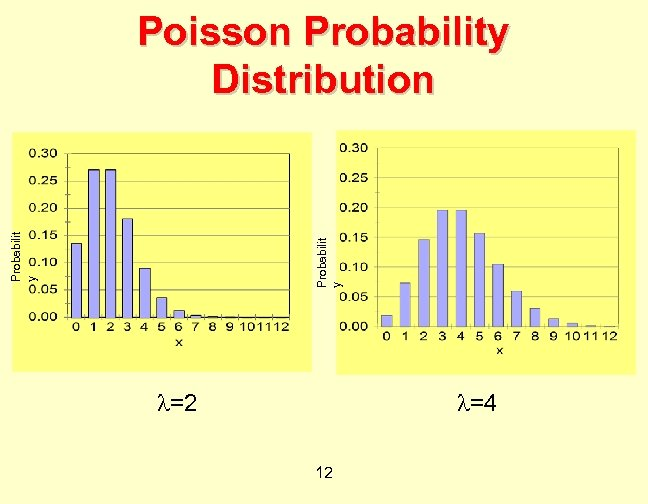Probabilit y Poisson Probability Distribution =2 =4 12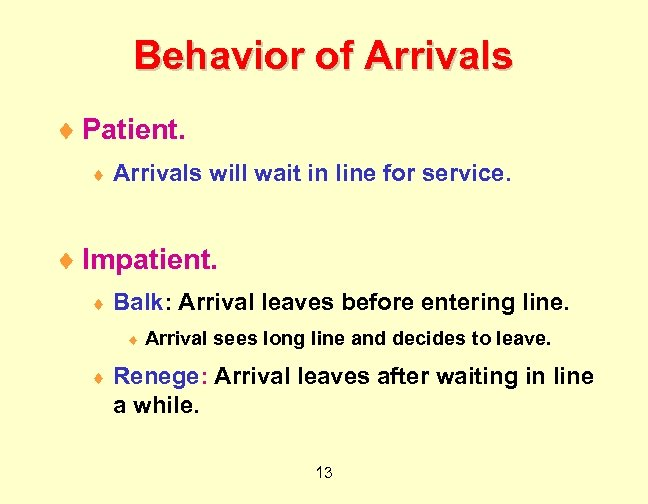Behavior of Arrivals ¨ Patient. ¨ Arrivals will wait in line for service. ¨ Impatient. ¨ Balk: Arrival leaves before entering line. ¨ ¨ Arrival sees long line and decides to leave. Renege: Arrival leaves after waiting in line a while. 13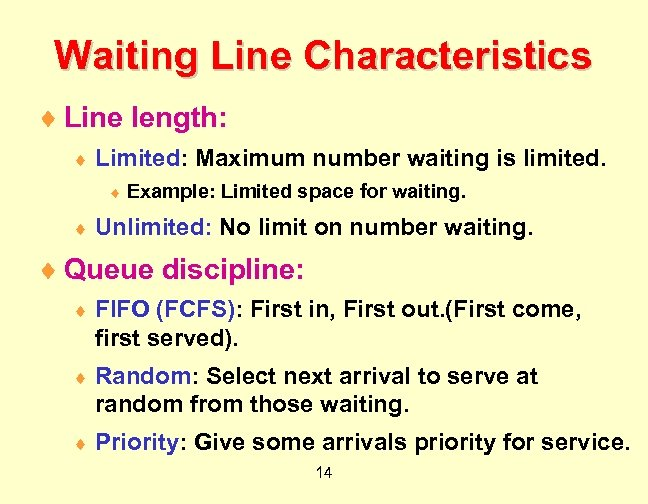Waiting Line Characteristics ¨ Line length: ¨ Limited: Maximum number waiting is limited. ¨ ¨ Example: Limited space for waiting. Unlimited: No limit on number waiting. ¨ Queue discipline: ¨ FIFO (FCFS): First in, First out. (First come, first served). ¨ Random: Select next arrival to serve at random from those waiting. ¨ Priority: Give some arrivals priority for service. 14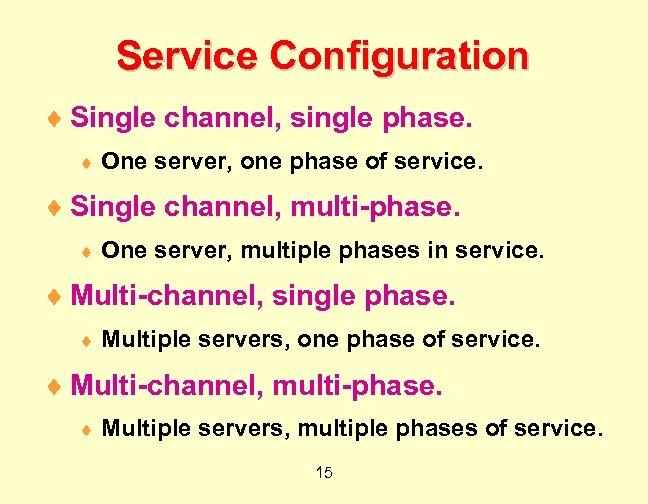Service Configuration ¨ Single channel, single phase. ¨ One server, one phase of service. ¨ Single channel, multi-phase. ¨ One server, multiple phases in service. ¨ Multi-channel, single phase. ¨ Multiple servers, one phase of service. ¨ Multi-channel, multi-phase. ¨ Multiple servers, multiple phases of service. 15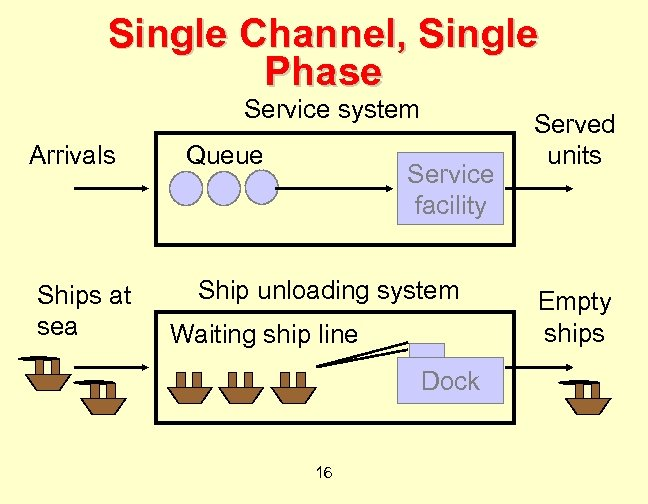Single Channel, Single Phase Service system Arrivals Ships at sea Queue Service facility Ship unloading system Waiting ship line Dock 16 Served units Empty ships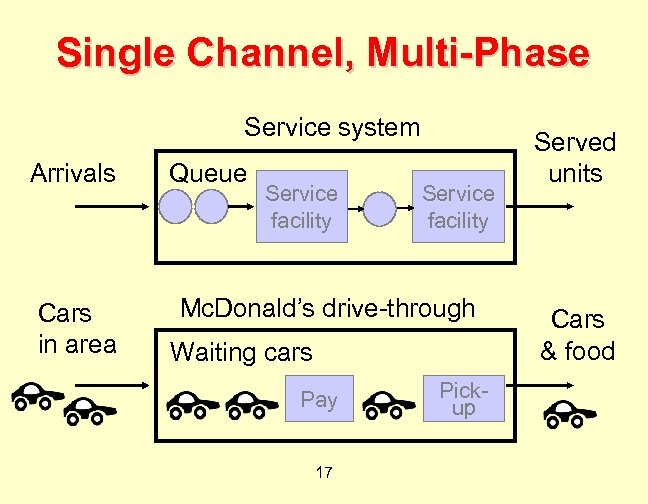Single Channel, Multi-Phase Service system Arrivals Cars in area Queue Service facility Mc. Donald’s drive-through Waiting cars Pay 17 Pickup Served units Cars & food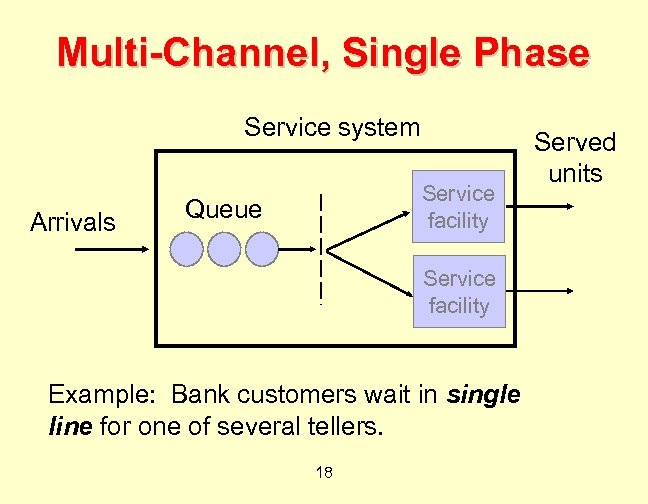Multi-Channel, Single Phase Service system Arrivals Service facility Queue Service facility Example: Bank customers wait in single line for one of several tellers. 18 Served units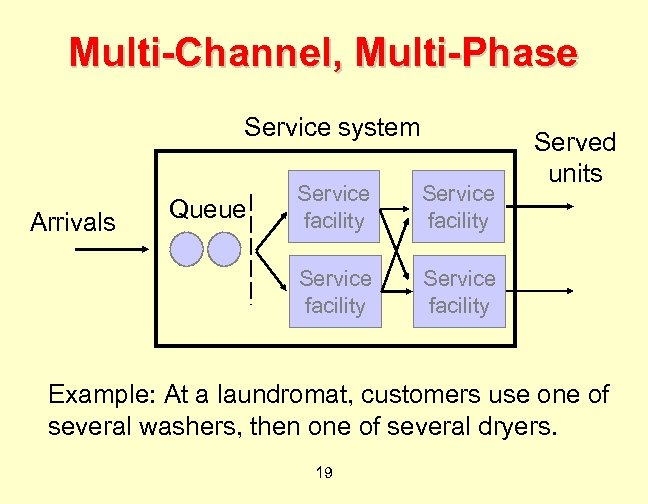Multi-Channel, Multi-Phase Service system Queue Service facility Arrivals Service facility Served units Service facility Example: At a laundromat, customers use one of several washers, then one of several dryers. 19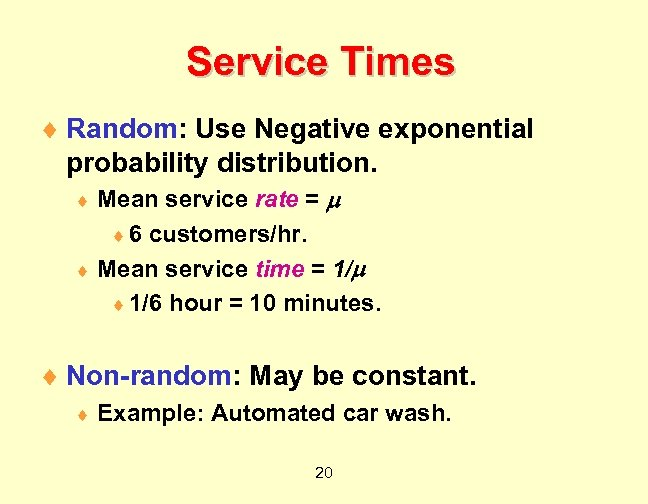Service Times ¨ Random: Use Negative exponential probability distribution. Mean service rate = ¨ 6 customers/hr. ¨ Mean service time = 1/ ¨ 1/6 hour = 10 minutes. ¨ ¨ Non-random: May be constant. ¨ Example: Automated car wash. 20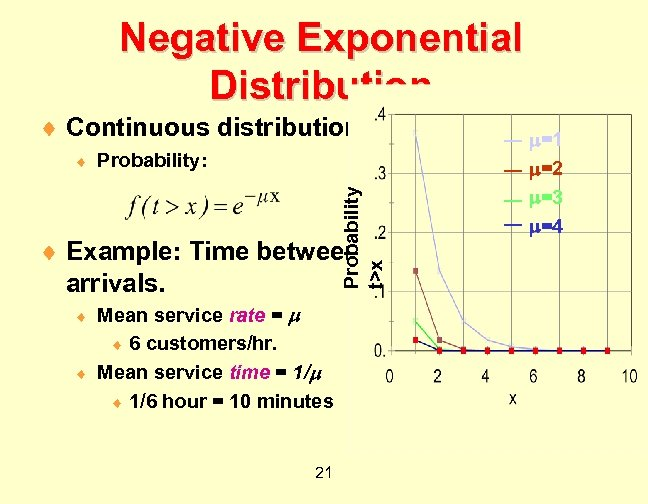Negative Exponential Distribution ¨ Continuous distribution. Probability: Probability t>x ¨ ¨ Example: Time between arrivals. Mean service rate = ¨ 6 customers/hr. ¨ Mean service time = 1/ ¨ 1/6 hour = 10 minutes ¨ 21 =1 =2 =3 =4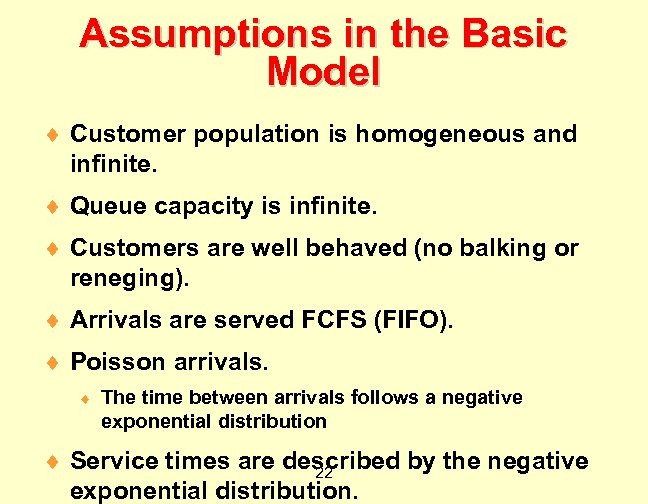Assumptions in the Basic Model ¨ Customer population is homogeneous and infinite. ¨ Queue capacity is infinite. ¨ Customers are well behaved (no balking or reneging). ¨ Arrivals are served FCFS (FIFO). ¨ Poisson arrivals. ¨ The time between arrivals follows a negative exponential distribution ¨ Service times are described by the negative 22 exponential distribution.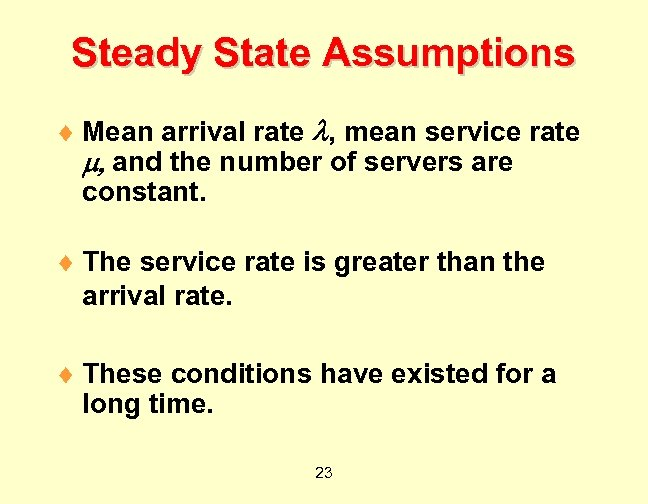Steady State Assumptions ¨ Mean arrival rate , mean service rate , and the number of servers are constant. ¨ The service rate is greater than the arrival rate. ¨ These conditions have existed for a long time. 23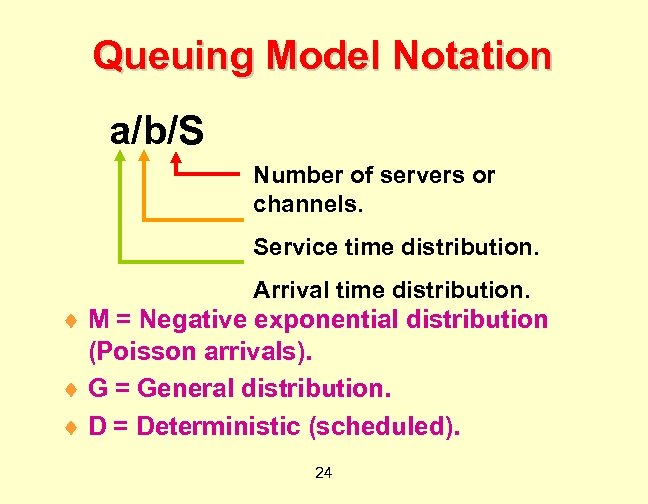Queuing Model Notation a/b/S Number of servers or channels. Service time distribution. Arrival time distribution. ¨ M = Negative exponential distribution (Poisson arrivals). ¨ G = General distribution. ¨ D = Deterministic (scheduled). 24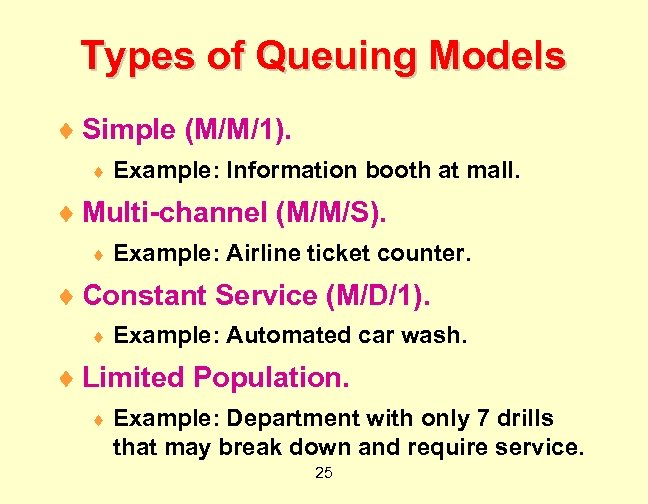Types of Queuing Models ¨ Simple (M/M/1). ¨ Example: Information booth at mall. ¨ Multi-channel (M/M/S). ¨ Example: Airline ticket counter. ¨ Constant Service (M/D/1). ¨ Example: Automated car wash. ¨ Limited Population. ¨ Example: Department with only 7 drills that may break down and require service. 25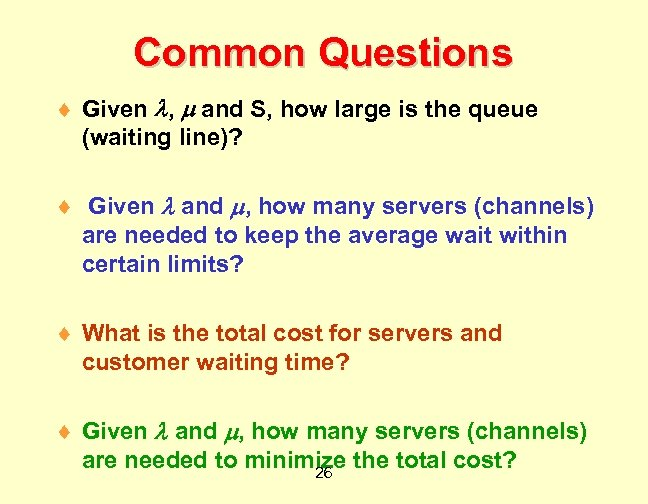Common Questions ¨ Given , and S, how large is the queue (waiting line)? ¨ Given and , how many servers (channels) are needed to keep the average wait within certain limits? ¨ What is the total cost for servers and customer waiting time? ¨ Given and , how many servers (channels) are needed to minimize the total cost? 26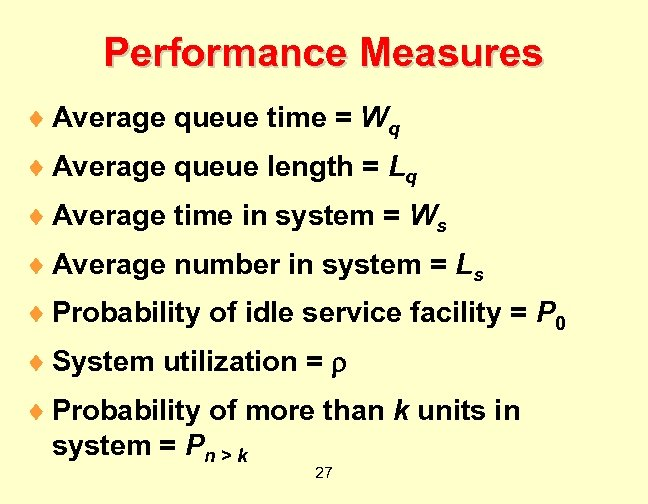Performance Measures ¨ Average queue time = Wq ¨ Average queue length = Lq ¨ Average time in system = Ws ¨ Average number in system = Ls ¨ Probability of idle service facility = P 0 ¨ System utilization = ¨ Probability of more than k units in system = Pn > k 27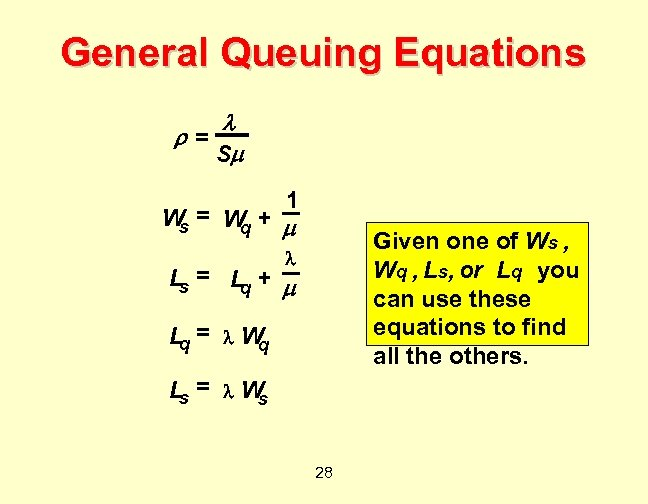General Queuing Equations = S 1 = W + W s q Given one of Ws , Wq , Ls, or Lq you can use these equations to find all the others. Ls = Lq + Lq = W q Ls = W s 28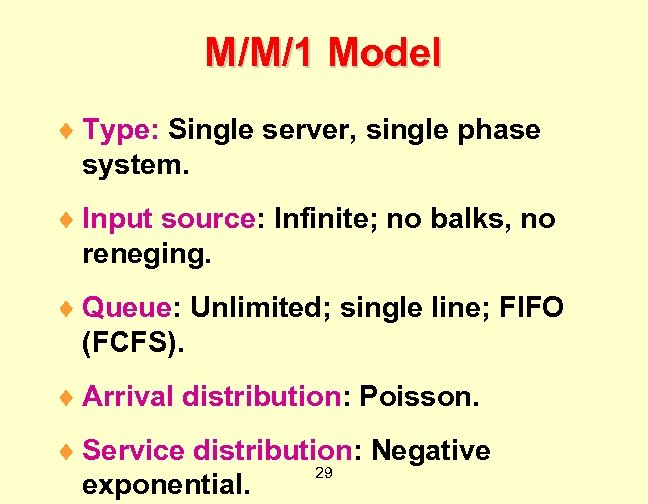M/M/1 Model ¨ Type: Single server, single phase system. ¨ Input source: Infinite; no balks, no reneging. ¨ Queue: Unlimited; single line; FIFO (FCFS). ¨ Arrival distribution: Poisson. ¨ Service distribution: Negative 29 exponential.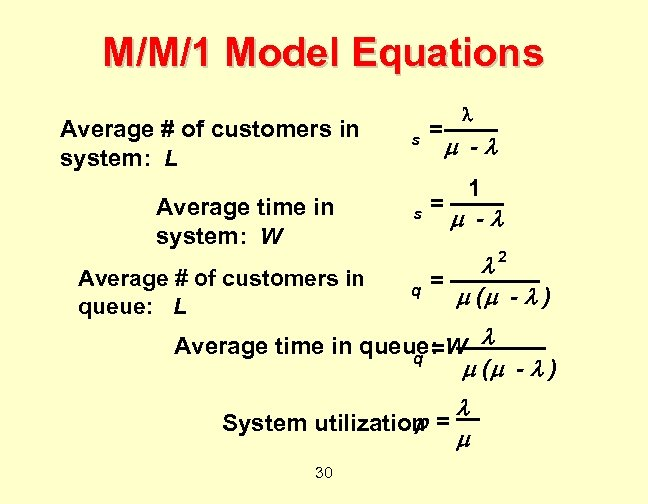M/M/1 Model Equations Average # of customers in system: L Average time in system: W Average # of customers in queue: L s = - 1 - 2 q = ( - ) Average time in queue: W q = ( - ) System utilization = 30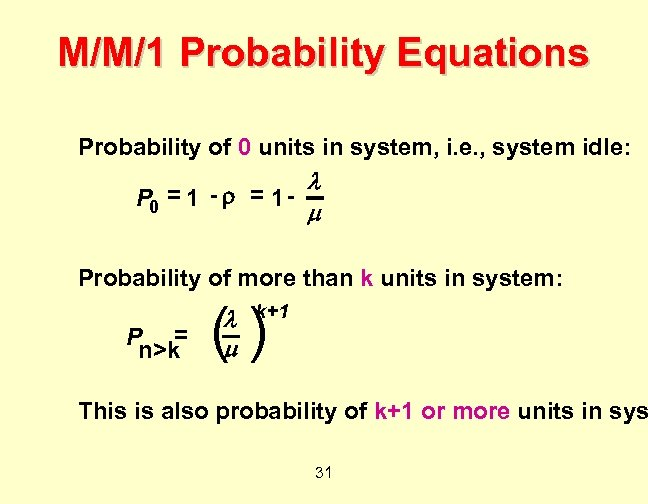M/M/1 Probability Equations Probability of 0 units in system, i. e. , system idle: P 0 = 1 - Probability of more than k units in system: P = n>k () k+1 This is also probability of k+1 or more units in sys 31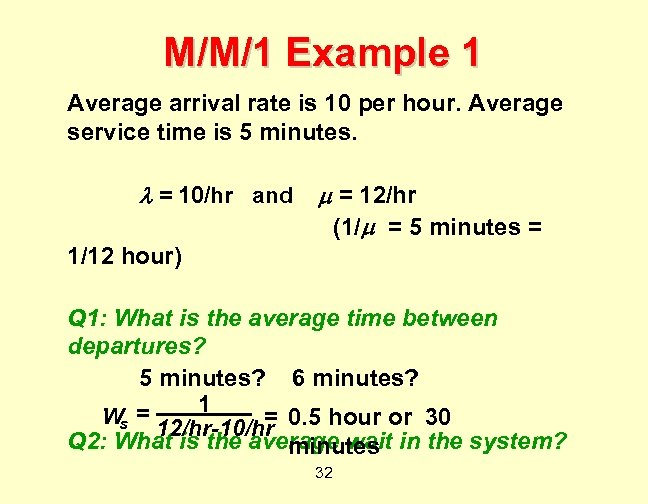M/M/1 Example 1 Average arrival rate is 10 per hour. Average service time is 5 minutes. = 10/hr and = 12/hr (1/ = 5 minutes = 1/12 hour) Q 1: What is the average time between departures? 5 minutes? 6 minutes? 1 W = = s 12/hr-10/hr 0. 5 hour or 30 Q 2: What is the average wait in the system? minutes 32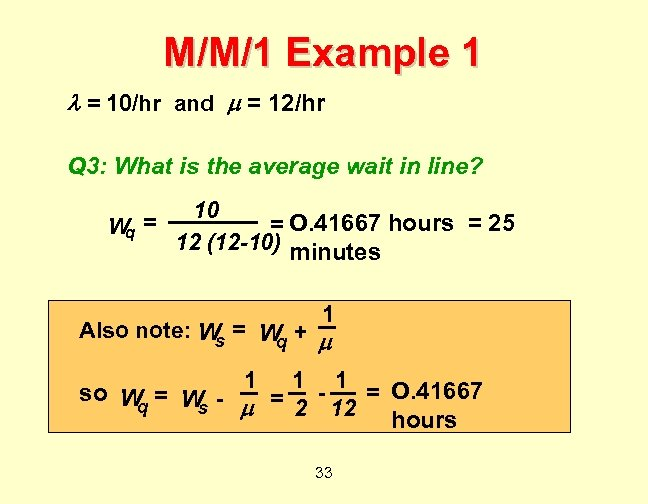M/M/1 Example 1 = 10/hr and = 12/hr Q 3: What is the average wait in line? W = q 10 = O. 41667 hours = 25 12 (12 -10) minutes 1 Also note: W = W + s q 1 1 1 so W = 2 - 12 = O. 41667 q s hours 33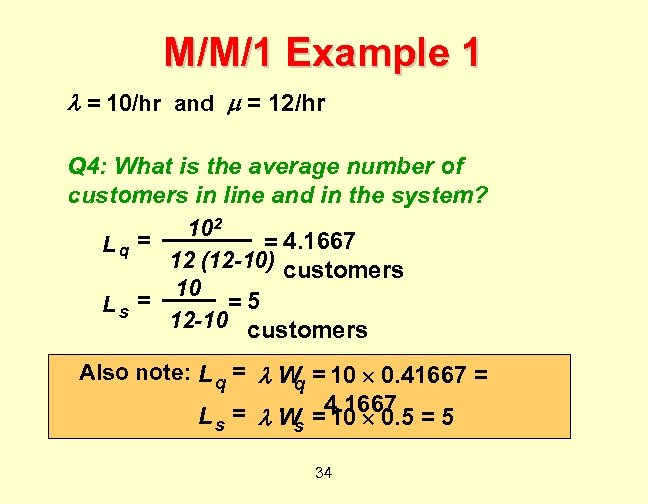M/M/1 Example 1 = 10/hr and = 12/hr Q 4: What is the average number of customers in line and in the system? 102 = 4. 1667 Lq = 12 (12 -10) customers 10 = =5 Ls 12 -10 customers Also note: L q = W = 10 0. 41667 = q 4. 1667 L s = W = 10 0. 5 = 5 s 34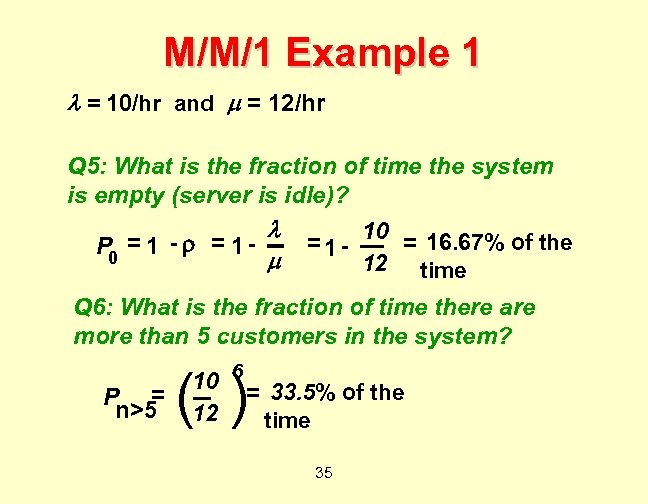M/M/1 Example 1 = 10/hr and = 12/hr Q 5: What is the fraction of time the system is empty (server is idle)? P 0 = 1 - 10 = 16. 67% of the 12 time Q 6: What is the fraction of time there are more than 5 customers in the system? P = n>5 10 6 = 33. 5% of the 12 time ( ) 35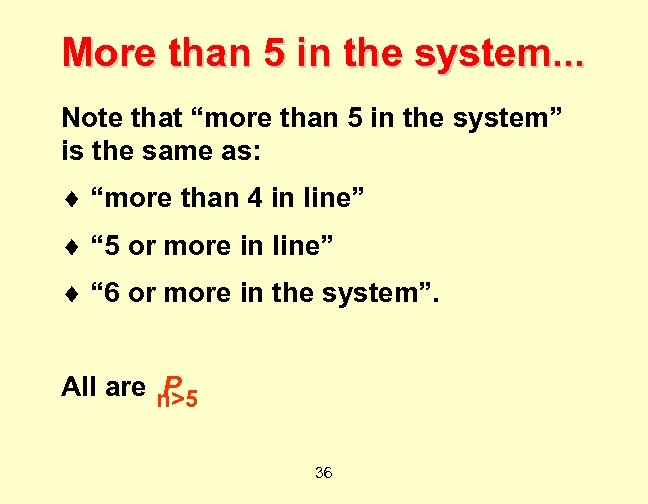More than 5 in the system. . . Note that “more than 5 in the system” is the same as: ¨ “more than 4 in line” ¨ “ 5 or more in line” ¨ “ 6 or more in the system”. All are n>5 P 36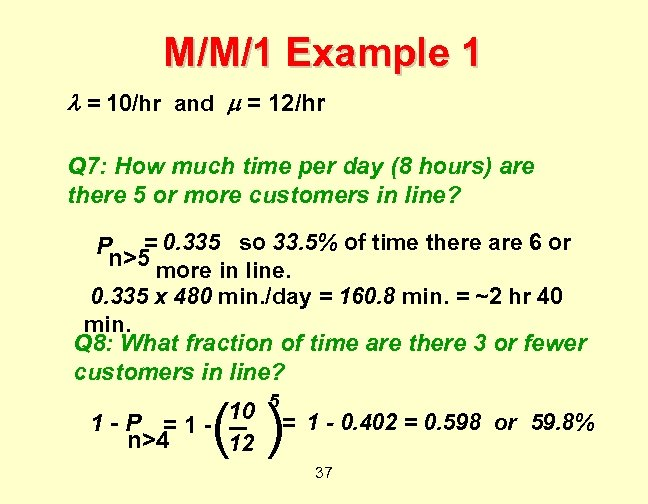M/M/1 Example 1 = 10/hr and = 12/hr Q 7: How much time per day (8 hours) are there 5 or more customers in line? P = 0. 335 so 33. 5% of time there are 6 or n>5 more in line. 0. 335 x 480 min. /day = 160. 8 min. = ~2 hr 40 min. Q 8: What fraction of time are there 3 or fewer customers in line? 10 5 = 1 - 0. 402 = 0. 598 or 59. 8% 1 -P =1 n>4 12 ( ) 37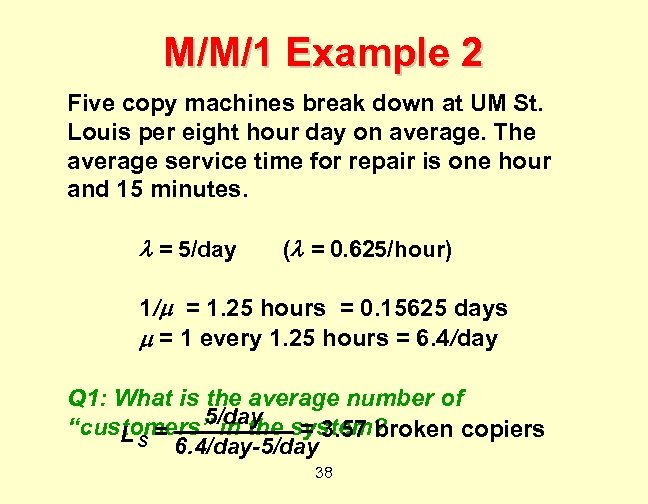M/M/1 Example 2 Five copy machines break down at UM St. Louis per eight hour day on average. The average service time for repair is one hour and 15 minutes. = 5/day ( = 0. 625/hour) 1/ = 1. 25 hours = 0. 15625 days = 1 every 1. 25 hours = 6. 4/day Q 1: What is the average number of 5/day “customers” in the system? = 3. 57 broken copiers = LS 6. 4/day-5/day 38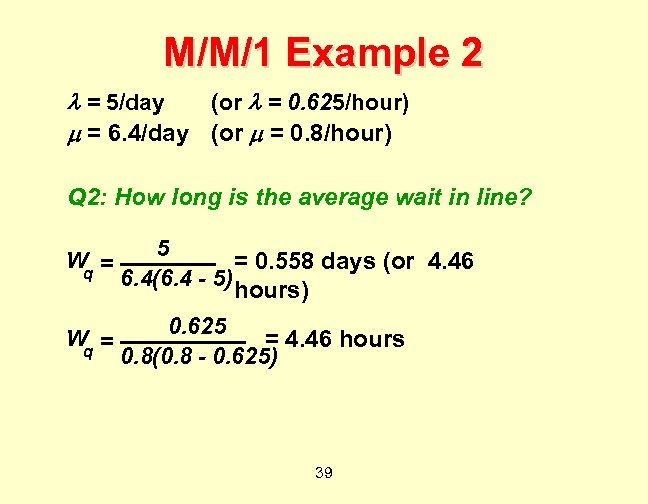M/M/1 Example 2 = 5/day (or = 0. 625/hour) = 6. 4/day (or = 0. 8/hour) Q 2: How long is the average wait in line? W = q 5 = 0. 558 days (or 4. 46 6. 4(6. 4 - 5) hours) W = q 0. 625 = 4. 46 hours 0. 8(0. 8 - 0. 625) 39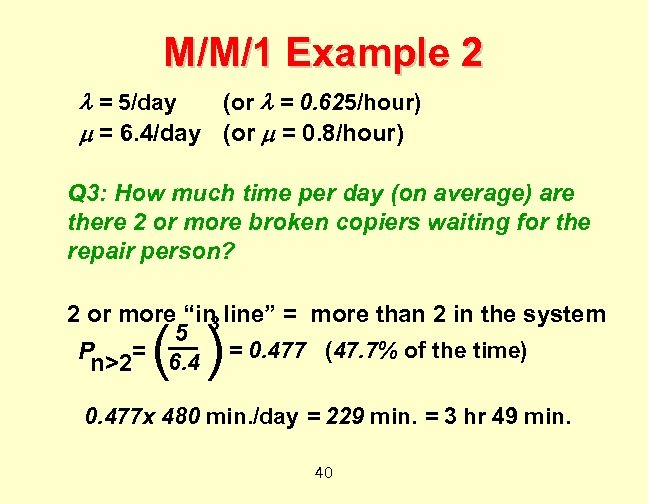M/M/1 Example 2 = 5/day (or = 0. 625/hour) = 6. 4/day (or = 0. 8/hour) Q 3: How much time per day (on average) are there 2 or more broken copiers waiting for the repair person? 2 or more “in line” = more than 2 in the system 5 3 = 0. 477 (47. 7% of the time) P n>2= 6. 4 ( ) 0. 477 x 480 min. /day = 229 min. = 3 hr 49 min. 40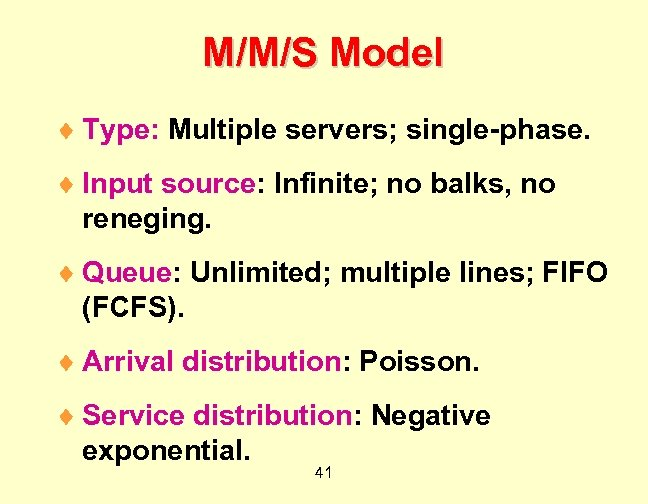M/M/S Model ¨ Type: Multiple servers; single-phase. ¨ Input source: Infinite; no balks, no reneging. ¨ Queue: Unlimited; multiple lines; FIFO (FCFS). ¨ Arrival distribution: Poisson. ¨ Service distribution: Negative exponential. 41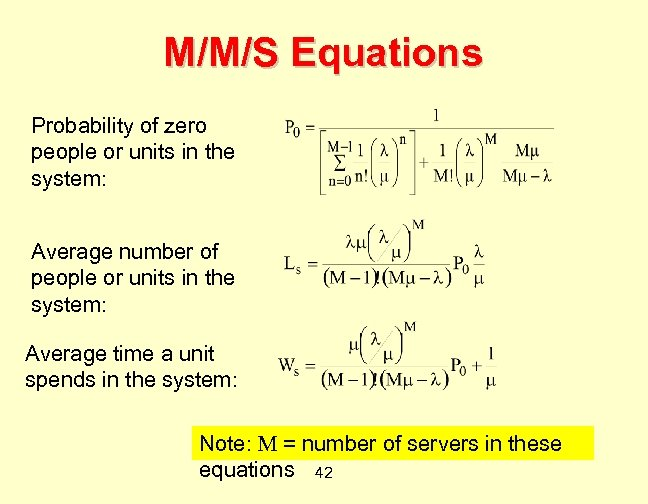M/M/S Equations Probability of zero people or units in the system: Average number of people or units in the system: Average time a unit spends in the system: Note: M = number of servers in these equations 42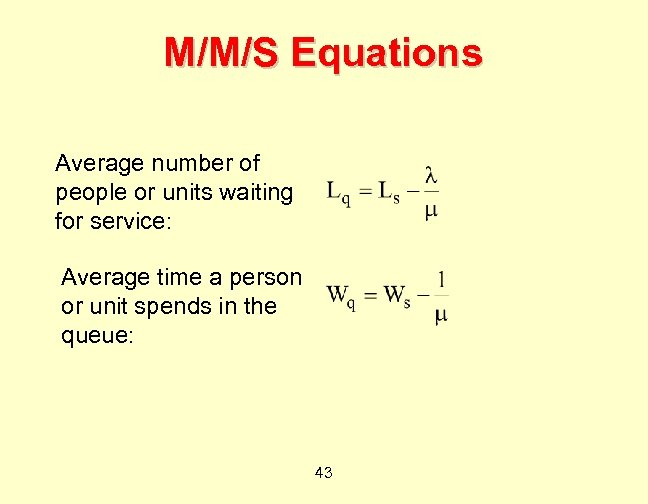M/M/S Equations Average number of people or units waiting for service: Average time a person or unit spends in the queue: 43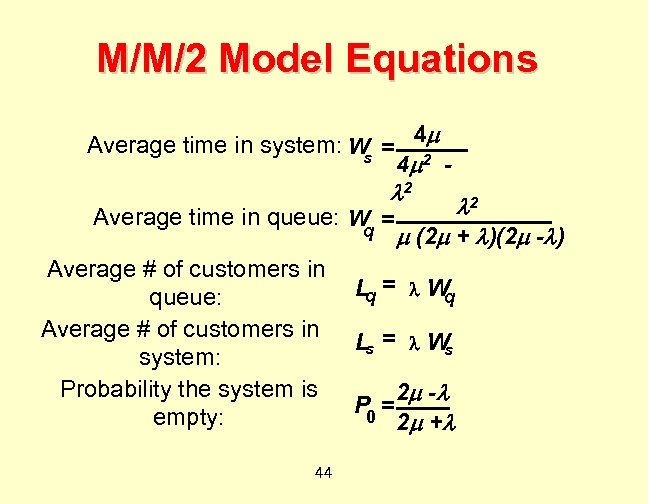M/M/2 Model Equations Average time in system: W = 4 s 4 2 2 2 Average time in queue: W = q (2 + )(2 - ) Average # of customers in Lq = W q queue: Average # of customers in Ls = W s system: Probability the system is 2 - P 0 = empty: 2 + 44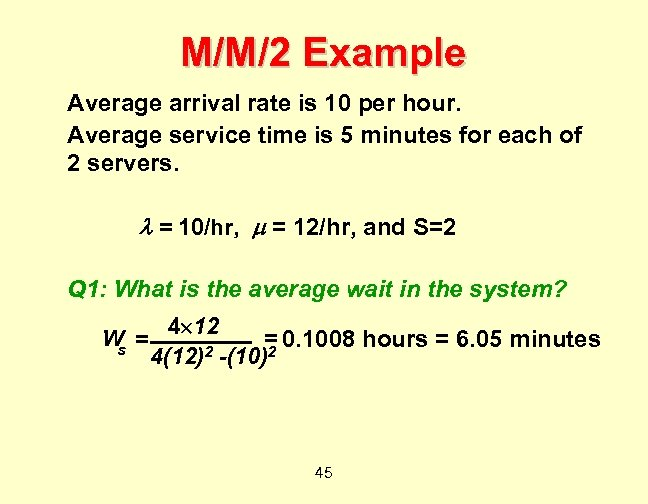M/M/2 Example Average arrival rate is 10 per hour. Average service time is 5 minutes for each of 2 servers. = 10/hr, = 12/hr, and S=2 Q 1: What is the average wait in the system? W = s 4 12 = 0. 1008 hours = 6. 05 minutes 2 -(10)2 4(12) 45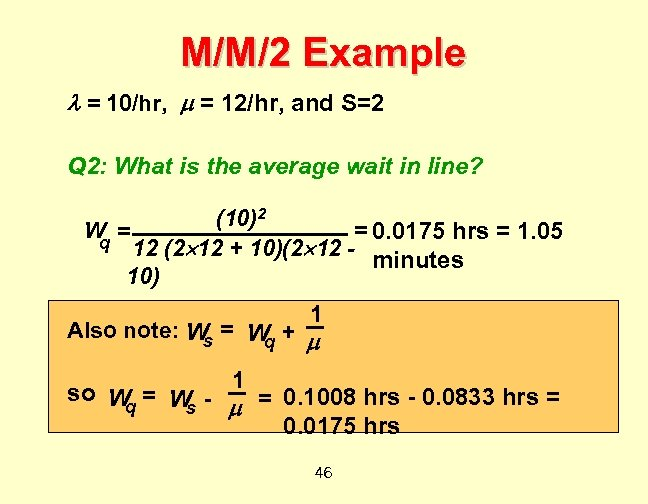M/M/2 Example = 10/hr, = 12/hr, and S=2 Q 2: What is the average wait in line? (10)2 W = = 0. 0175 hrs = 1. 05 q 12 (2 12 + 10)(2 12 minutes 10) 1 Also note: W = W + s q 1 so W = W q s = 0. 1008 hrs - 0. 0833 hrs = 0. 0175 hrs 46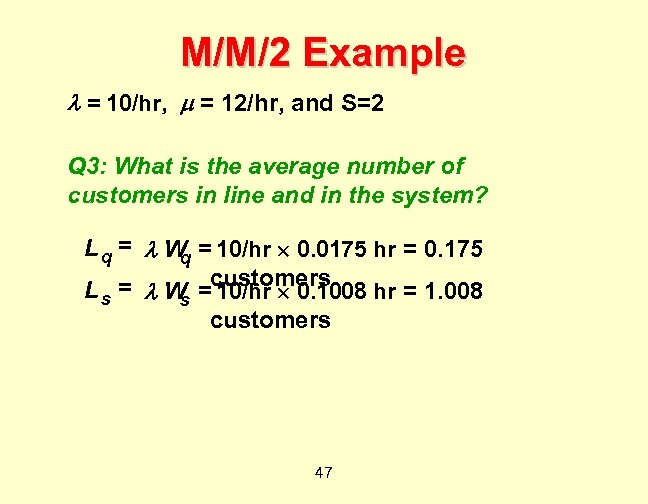M/M/2 Example = 10/hr, = 12/hr, and S=2 Q 3: What is the average number of customers in line and in the system? L q = W = 10/hr 0. 0175 hr = 0. 175 q customers L s = W = 10/hr 0. 1008 hr = 1. 008 s customers 47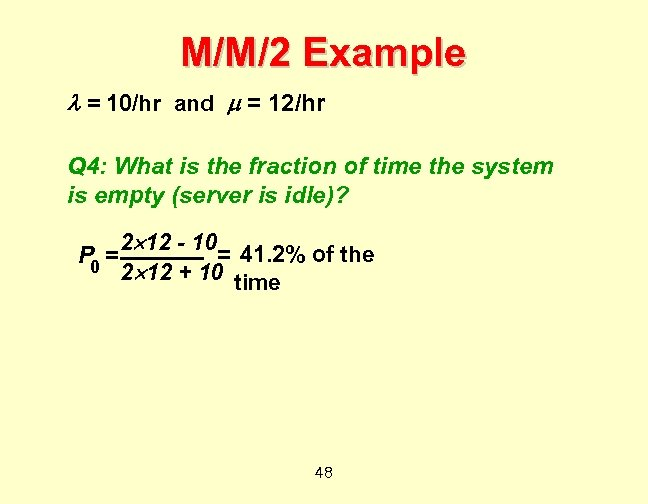M/M/2 Example = 10/hr and = 12/hr Q 4: What is the fraction of time the system is empty (server is idle)? P 0 = 2 12 - 10 = 41. 2% of the 2 12 + 10 time 48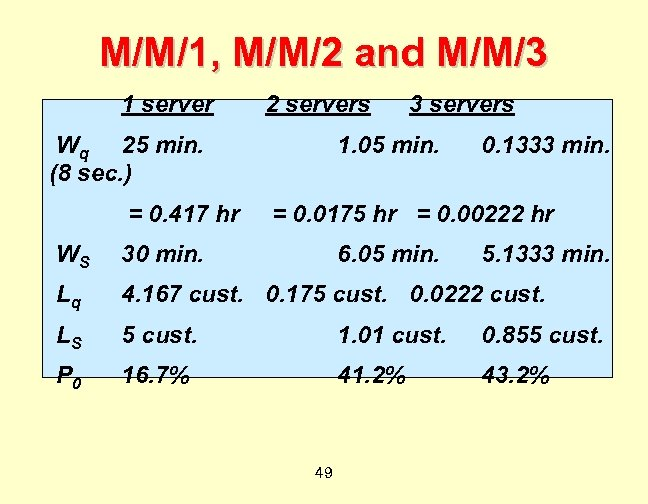M/M/1, M/M/2 and M/M/3 1 server 2 servers Wq 25 min. (8 sec. ) = 0. 417 hr 3 servers 1. 05 min. 0. 1333 min. = 0. 0175 hr = 0. 00222 hr WS 30 min. 6. 05 min. Lq 4. 167 cust. 0. 175 cust. 0. 0222 cust. LS 5 cust. 1. 01 cust. 0. 855 cust. P 0 16. 7% 41. 2% 43. 2% 49 5. 1333 min.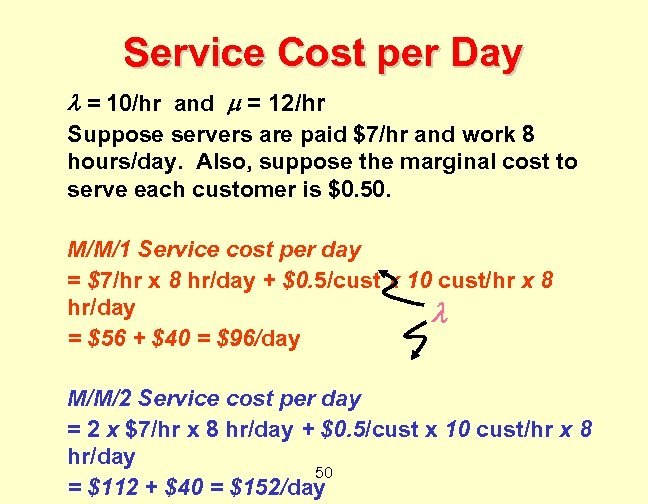Service Cost per Day = 10/hr and = 12/hr Suppose servers are paid \$7/hr and work 8 hours/day. Also, suppose the marginal cost to serve each customer is \$0. 50. M/M/1 Service cost per day = \$7/hr x 8 hr/day + \$0. 5/cust x 10 cust/hr x 8 hr/day = \$56 + \$40 = \$96/day M/M/2 Service cost per day = 2 x \$7/hr x 8 hr/day + \$0. 5/cust x 10 cust/hr x 8 hr/day 50 = \$112 + \$40 = \$152/day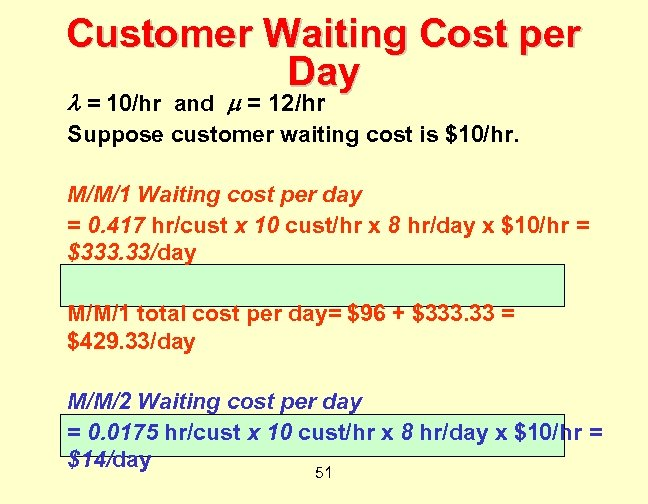Customer Waiting Cost per Day = 10/hr and = 12/hr Suppose customer waiting cost is \$10/hr. M/M/1 Waiting cost per day = 0. 417 hr/cust x 10 cust/hr x 8 hr/day x \$10/hr = \$333. 33/day M/M/1 total cost per day= \$96 + \$333. 33 = \$429. 33/day M/M/2 Waiting cost per day = 0. 0175 hr/cust x 10 cust/hr x 8 hr/day x \$10/hr = \$14/day 51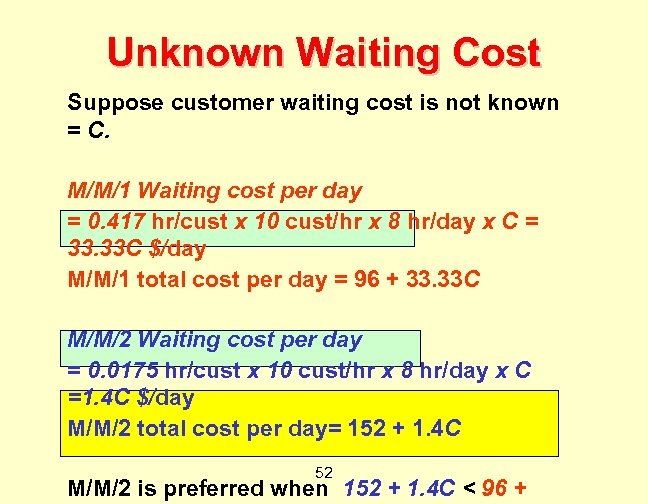Unknown Waiting Cost Suppose customer waiting cost is not known = C. M/M/1 Waiting cost per day = 0. 417 hr/cust x 10 cust/hr x 8 hr/day x C = 33. 33 C \$/day M/M/1 total cost per day = 96 + 33. 33 C M/M/2 Waiting cost per day = 0. 0175 hr/cust x 10 cust/hr x 8 hr/day x C =1. 4 C \$/day M/M/2 total cost per day= 152 + 1. 4 C 52 M/M/2 is preferred when 152 + 1. 4 C < 96 +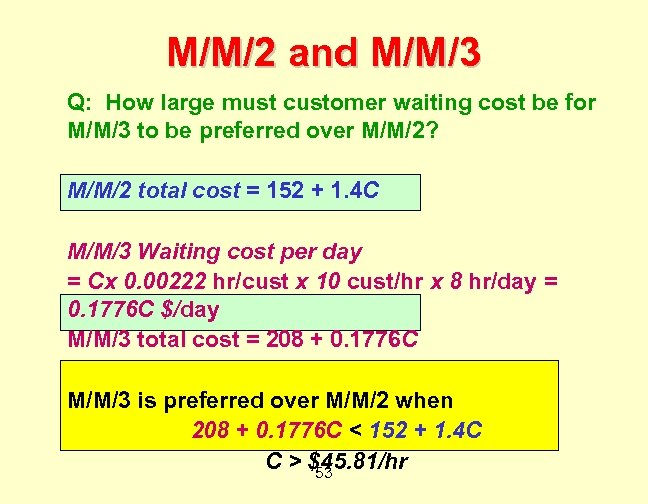M/M/2 and M/M/3 Q: How large must customer waiting cost be for M/M/3 to be preferred over M/M/2? M/M/2 total cost = 152 + 1. 4 C M/M/3 Waiting cost per day = Cx 0. 00222 hr/cust x 10 cust/hr x 8 hr/day = 0. 1776 C \$/day M/M/3 total cost = 208 + 0. 1776 C M/M/3 is preferred over M/M/2 when 208 + 0. 1776 C < 152 + 1. 4 C C > \$45. 81/hr 53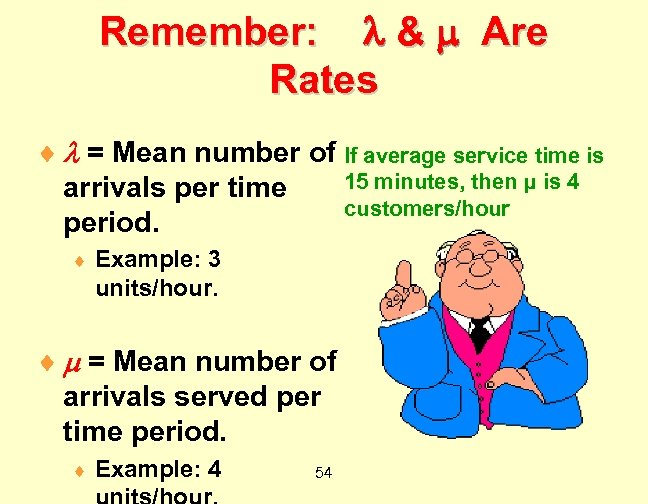Remember: & Are Rates ¨ = Mean number of If average service time is 15 minutes, then μ is 4 arrivals per time customers/hour period. ¨ Example: 3 units/hour. ¨ = Mean number of arrivals served per time period. ¨ Example: 4 54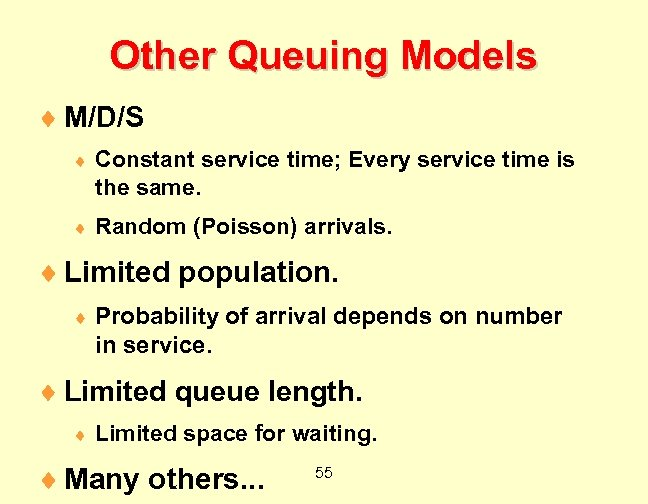Other Queuing Models ¨ M/D/S ¨ Constant service time; Every service time is the same. ¨ Random (Poisson) arrivals. ¨ Limited population. ¨ Probability of arrival depends on number in service. ¨ Limited queue length. ¨ Limited space for waiting. ¨ Many others. . . 55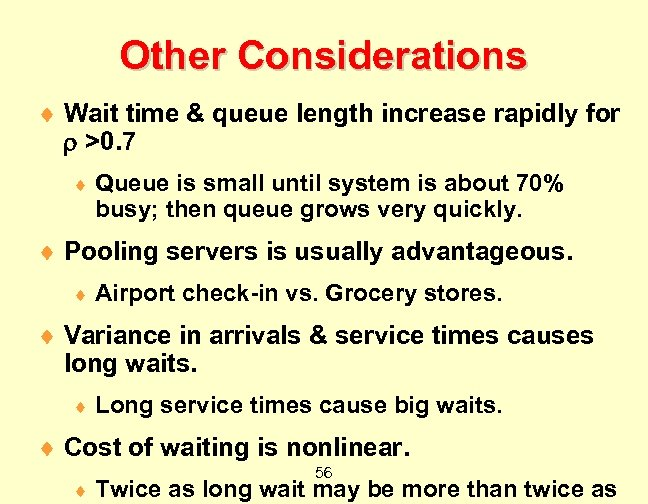Other Considerations ¨ Wait time & queue length increase rapidly for >0. 7 ¨ Queue is small until system is about 70% busy; then queue grows very quickly. ¨ Pooling servers is usually advantageous. ¨ Airport check-in vs. Grocery stores. ¨ Variance in arrivals & service times causes long waits. ¨ Long service times cause big waits. ¨ Cost of waiting is nonlinear. ¨ 56 Twice as long wait may be more than twice as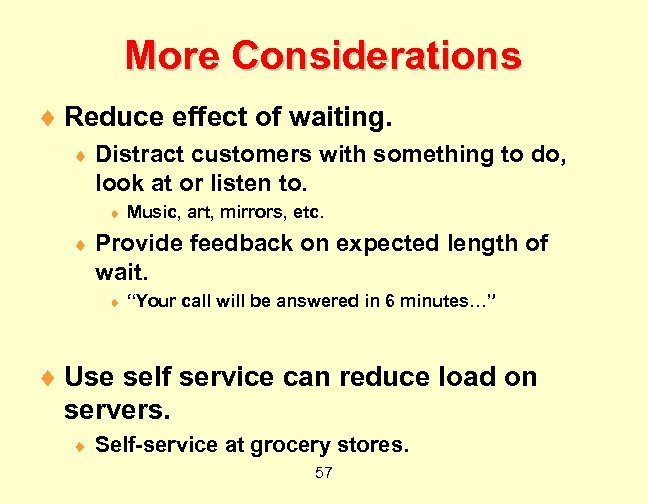More Considerations ¨ Reduce effect of waiting. ¨ Distract customers with something to do, look at or listen to. ¨ ¨ Music, art, mirrors, etc. Provide feedback on expected length of wait. ¨ “Your call will be answered in 6 minutes…” ¨ Use self service can reduce load on servers. ¨ Self-service at grocery stores. 57